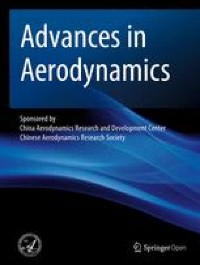# Extension of the KDO turbulence/transition model to account for roughness – Advances in Aerodynamics

#### ByChunfei Fang and Jinglei Xu

Jan 14, 2022### Baseline model

The Kinetic energy Dependent Only model (KDO)  adopts a physics-based formulation, with less empiricism, for Reynolds Averaged Navier–Stokes Equation closure. For transition predictions, KDO does not explicitly model a specific transition mechanism but can capture many types of transition phenomena. Along with the evolution of the turbulent kinetic energy (k) equation, transition phenomena naturally appear, which is similar to what Navier–Stokes equations do. The key is that all the model elements for the RANS closure are flow-structure-adaptive. The model equations read,

$${k}_{,t}+{left({U}_jkright)}_{,j}=-overline{u_i^{prime }{u}_j^{prime }}{U}_{i,j}+{left[left(nu +{nu}_tright){k}_{,j}right]}_{,j}-varepsilon$$

(1)

$$varepsilon ={varepsilon}_1+{varepsilon}_2$$

(2)

$${varepsilon}_1=2nu {sqrt{k}}_{,j}{sqrt{k}}_{,j}$$

(3)

The turbulence Reynolds number, Rek can be used for model calibrations. Reis defined as ({Re}_k=sqrt{k}d/nu), in which d is the wall distance. It is obvious that Rek is a local variable and such a variable is beneficial to parallel computation.

For Rek < 10,

$${varepsilon}_2={A}_{varepsilon }{k}^{3/2}/d$$

(4)

$${A}_{varepsilon }=1.34{left({Re}_k/0.25right)}^{-0.8}{left(1+{left({Re}_k/0.25right)}^{1.5}right)}^{0.45/1.5}{left(1+{left({Re}_k/2.4right)}^{1.5}right)}^{-0.1/1.5}$$

(5)

For Rek ≥ 10,

$${varepsilon}_2=min left({A}_{varepsilon },0.8right){k}^{3/2}max left(1/L,left(1-{mathrm{e}}^{-{Re}_k/1300}right)/dright)$$

(6)

$${displaystyle begin{array}{l}{A}_{varepsilon }=1.4{left({Re}_k/4.3right)}^{-1.9}{left(1+{left({Re}_k/4.3right)}^5right)}^{0.2/5}{left(1+{left({Re}_k/28right)}^{4.9}right)}^{2.76/4.9}\ {}{left(1+{left({Re}_k/66right)}^{10}right)}^{-0.15/10}{left(1+{left({Re}_k/110right)}^{10}right)}^{0.12/10}{left(1+{left({Re}_k/175right)}^{10}right)}^{-0.4/10}end{array}}$$

(7)

$$L=sqrt{Omega_icdot {Omega}_i}/sqrt{nabla_j{Omega}_icdot {nabla}_j{Omega}_i},kern1em {Omega}_i=nabla times mathbf{U}$$

(8)

By extending Bradshaw’s assumption down to the wall, the Reynolds stress constitutive relation is established as,

$${R}_b={tau}_{12}/k$$

(9)

$$-overline{u_i^{prime }{u}_j^{prime }}={R}_bkfrac{2{S}_{ij}}{S},kern1em S=sqrt{2{S}_{ij}{S}_{ij}}$$

(10)

where τ12 is the principal Reynolds shear stress, (-overline{u_i^{prime }{u}_j^{prime }}) is the Reynolds stress tensor, k is the turbulent kinetic energy, Rb is the Bradshaw’s coefficient, and Sij is the mean strain rate tensor.

$${R}_b=min left[0.018{left({Re}_k/1right)}^{0.56}{left(1+{left({Re}_k/120right)}^{2.5}right)}^{-0.56/2.5}{left(1+{left({Re}_k/225right)}^{10}right)}^{0.05/10},0.283right]$$

(11)

in which, ({Re}_k=rho sqrt{k}d/mu) is the turbulent Reynolds number. For the KDO transition model , the Bradshaw’s coefficient reads,

$${R}_b=min left[0.1165{left(r/1right)}^{0.37}{left(1+{left(r/1right)}^{1.15}right)}^{-0.157/1.15}{left(1+{left(r/72right)}^2right)}^{-0.213/2},0.283right]$$

(12)

in which, μt/μ is eddy viscosity ratio. The eddy viscosity ratio, a measurement of the intensity of turbulence, is a transport variable. Due to the transport properties of r, Rb is capable of capturing transition phenomena, such as bypass transition, natural transition, separation induced transition and cross flow transition. To conclude, the KDO model is one turbulence model that could predict both fully turbulent flows and laminar-turbulent flow transitions, by solving only the k equation. The information of turbulence and laminar-turbulent flow transition is included in the k equation, and there is no such distinction as turbulent equation or intermittency equation. Therefore, a typical roughness correction imposed on the KDO model could potentially reflect the roughness effects on the transition process.

### Roughness extension

The idea of “equivalent sand grain approach” is employed here. The basic idea is that, according to experimental data , the log-law still holds in a turbulent boundary layer with wall roughness. The difference is that, the y+-U+ profile moves upwards, leading to a shifted log-law,

$${u}^{+}=frac{1}{kappa}ln frac{y{}^{+}}{h_s^{+}}+C$$

(13)

where hs is the equivalent sand grain height. In Eq. (13), y and hs are non-dimensioned by ν/uτ. For example, yequals yuτ/ν, in which ({u}_{tau }=sqrt{tau_w/rho }) is the wall friction velocity. The position of the wall can be considered to be raised from y to y+d0. d0, named as the equivalent roughness height, is the roughness height for CFD computations. d0 is less than hs due to that there are spaces among sand elements. On the other hand, roughness enhances the turbulent viscosity to a value that is much larger than the molecular viscosity on the wall, and we have,

$${u}_{tau}^2={nu}_tfrac{partial U}{partial y}={u}_{tau}kappa left(y+{d}_0right)frac{partial U}{partial y}$$

(14)

Note that Eq. (14) assumes that the boundary layer is fully turbulent, so Eq. (14) might not be valid for transitional flows. The solution of Eq. (14) is,

$${u}^{+}=frac{1}{kappa}left[ln left(y+{d}_0right)-ln left({d}_0right)right]$$

(15)

By substituting Eq. (13) to Eq. (15), it is easy to obtain the relationship between d0 and hs.

$${d}_0=exp left(- Ckappa right){h}_s$$

(16)

Aupoix and Spalart  set C to 8.5, which is valid for very rough surfaces. In such a roughness model, d0 equals 0.03hs, and this is inconsistent with the intuition that d0 and hs should be of the same magnitude. On the other hand, Chedevergne and Aupoix  stated that C can range from 5.5 to 9.7, indicating that even if the log-law still holds, the universality is compromised.

The present work employs the idea of “equivalent sand grain approach”, but the empirical coefficient is determined by reasoning instead of the shifted log-law. This work also employs the equivalent roughness height d0. The relation between d0 and hs is also

$${d}_0={C}_r{h}_s$$

(17)

If the real roughness height h is known,

The original wall distance, d, in the KDO turbulence model is replayed by,

$$tilde{d}=d+{d}_0$$

(19)

For a surface uniformly covered with sand grain, considering that the sand grain is with spherical shape, Cr is set to 0.35. For a surface that experienced polishing treatment, the rough elements are uniformly distributed on the surface, so ({C}_r^0) is around 0.5. The two constants are assessed in the following sections via CFD simulations.

The roughness effect is introduced via boundary conditions. The turbulent kinetic energy, k, on a smooth wall is zero. But for a rough wall, with the definition ({k}^{+}=k/{u}_{tau}^2),

$${k}_w={left.{k}^{+}right|}_w{u}_{tau}^2={left.{k}^{+}right|}_w{left.{}left(nu +{nu}_tright)frac{partial U}{partial y}right|}_w$$

(20)

k+ can be expressed by y+, and a model is calibrated by flat plate boundary layer at Reθ = 4060 ,

$${displaystyle begin{array}{l}{k}^{+}=fleft({y}^{+}right)=0.131{left({y}^{+}/1right)}^2{left(1+{left({y}^{+}/3right)}^{1.6}right)}^{-0.5/1.6}{left(1+{left({y}^{+}/8right)}^{3.9}right)}^{-1.38/3.9}\ {}kern8.5em {left(1+{left({y}^{+}/19right)}^{5.4}right)}^{-0.46/5.4}{left(1+{left({y}^{+}/40right)}^4right)}^{0.18/4}end{array}}$$

(21)

As seen in Fig. 1, Eq. (21) is valid in the range 0 < y+ (1) < 103, which covers the viscous layer, the buffer layer, and the log layer. Along with the increment of y+, the flow undergoes laminar state, laminar-turbulent transition, and turbulent state, indicating that Eq. (21) could potentially capture all the flow states. For a rough wall, k+|w in Eq. (20) is calculated by,

$${displaystyle begin{array}{l}{left.{k}^{+}right|}_w=0.131{left({d}_0^{+}/1right)}^2{left(1+{left({d}_0^{+}/3right)}^{1.6}right)}^{-0.5/1.6}{left(1+{left({d}_0^{+}/8right)}^{3.9}right)}^{-1.38/3.9}\ {}kern8.5em {left(1+{left({d}_0^{+}/19right)}^{5.4}right)}^{-0.46/5.4}{left(1+{left({d}_0^{+}/40right)}^4right)}^{0.18/4}end{array}}$$

(22)

On a smooth wall νt|w is set to 0. As to νt|w in Eq. (20), a Neumann boundary condition is employed,

$${left.frac{partial {nu}_t}{partial n}right|}_w=frac{nu_t}{tilde{d}}$$

(23)

The KDO RANS model  with this roughness correction is termed as KDOR. The KDO transition model  (KDO-tran) with this roughness correction is termed as KDOR-tran.